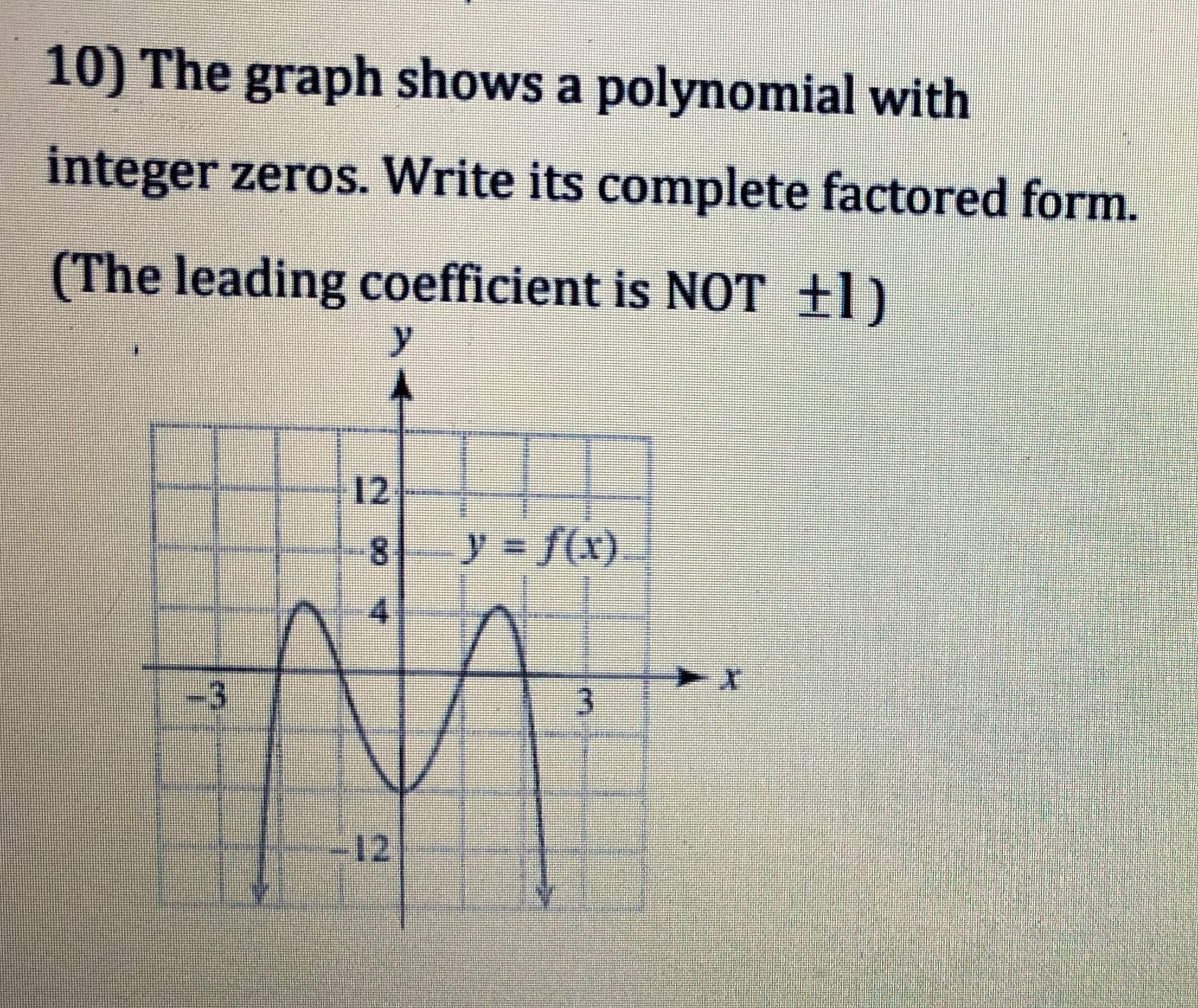# 10) The graph shows a polynomial withinteger zeros. Write its complete factored form.(The leading coefficient is NOT +1)(1

Question
9 viewshelp_outlineImage Transcriptionclose10) The graph shows a polynomial with integer zeros. Write its complete factored form. (The leading coefficient is NOT +1) (1 fullscreen
check_circle

Step 1

Result used:

The Factor Theorem:

Step 2

From the graph, it is observed that, the functional value is zero at x = −2, −1, 1 and 2.

Step 3

Obtain the value a as follows.

From the graph, it is observed that...

### Want to see the full answer?

See Solution

#### Want to see this answer and more?

Solutions are written by subject experts who are available 24/7. Questions are typically answered within 1 hour.*

See Solution
*Response times may vary by subject and question.
Tagged in

### Polynomials